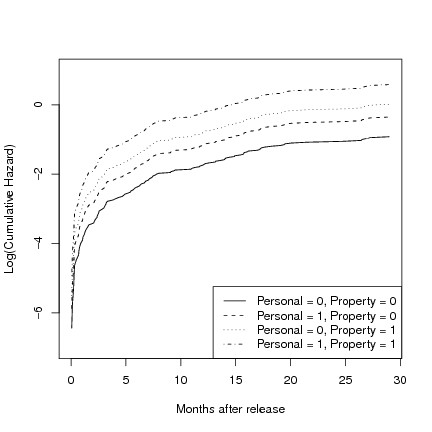DATA MINING
Desktop Survival Guide
by Graham Williams# Example from Singer and Willett

Obtain the data:

 ```> library(survival) > rearrest<-read.table("http://www.ats.ucla.edu/stat/examples/alda/rearrest.csv", sep=",", header=T) ```

Separate data into two datasets, grouped on personal. Next, run an empty Cox model and use the survfit function to generate the event times and estimated survival probabilities at the event times for both datasets. These probabilities are plotted, using a step function. The plot represents the estimated survivor function for the recidivism data stratified by the predictor personal.

 ```> rearrest0 <- subset(rearrest, personal == 0) > rearrest1 <- subset(rearrest, personal == 1) > f14.1.0 <- summary(survfit(Surv(rearrest0\$months, abs(rearrest0\$censor - 1)) ~ 1)) > f14.1.1 <- summary(survfit(Surv(rearrest1\$months, abs(rearrest1\$censor - 1)) ~ 1)) > s.hat.0 <- f14.1.0[] > time.0 <- f14.1.0[] > s.hat.1 <- f14.1.1[] > time.1 <- f14.1.1[] > s.hat.steps.0 <- stepfun(time.0, c(1, s.hat.0)) > s.hat.steps.1 <- stepfun(time.1, c(1, s.hat.1)) > plot(s.hat.steps.0, do.points = FALSE, xlim = c(0, 36), ylim = c(0,1), ylab = "Estimated Survival", xlab = "Months after release", main = "") > lines(s.hat.steps.1, do.points = FALSE, xlim = c(0,36), lty = 2) > legend("bottomleft", c("Personal = 0", "Personal = 1"), lty = c(1, 2)) ```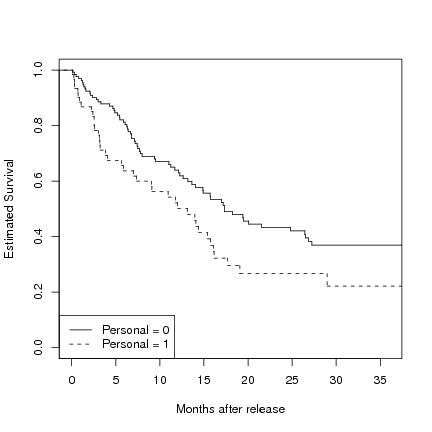Now graph the corresponding cumulative hazard stratified by personal, calculated by taking the negative log of the survival probabilities.

 ```> h.hat.0 <- -log(s.hat.0) > h.hat.1 <- -log(s.hat.1) > h.hat.steps.0 <- stepfun(time.0, c(0, h.hat.0)) > h.hat.steps.1 <- stepfun(time.1, c(0, h.hat.1)) > plot(h.hat.steps.0, do.points = FALSE, xlim = c(0, 36), ylim = c(0,1.4), ylab = "Estimated Cumulative Hazard", xlab = "Months after release", main = "") > lines(h.hat.steps.1, do.points = FALSE, xlim = c(0,36), lty = 2) > legend("bottomright", c("Personal = 0", "Personal = 1"), lty = c(1, 2)) ```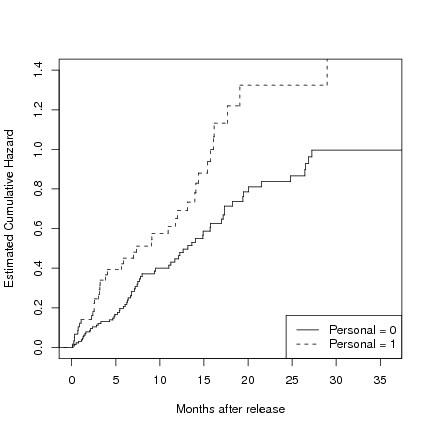Introduce a function, smooth, to take the time and estimated survival probabilities from survfit and aggregates the hazard over a given width of time. This function uses the same smoothing algorithm the book authors used. Using one of R's kernel smoothing functions (like ksmooth) would not exactly reproduce the graphs as they appear in the text. In this instance, the bandwidth of the graph in the text appears to be 8.

 ```> smooth<- function(width, time, survive){ n=length(time) lo=time + width hi=time[n] - width npt=50 inc=(hi-lo)/npt s=lo+t(c(1:npt))*inc slag = c(1, survive[1:n-1]) h=1-survive/slag x1 = as.vector(rep(1, npt))%*%(t(time)) x2 = t(s)%*%as.vector(rep(1,n)) x = (x1 - x2) / width k=.75*(1-x*x)*(abs(x)<=1) lambda=(k%*%h)/width smoothed= list(x = s, y = lambda) return(smoothed) } > bw1.0 <- smooth(8, time.0, s.hat.0) > bw1.1 <- smooth(8, time.1, s.hat.1) > plot(bw1.0\$x, bw1.0\$y, type = "l", xlim = c(0, 36), ylim = c(0, .08), xlab = "Months after release", ylab = "Smoothed hazard") > points(bw1.1\$x, bw1.1\$y, type = "l", lty = 2) > legend("bottomright", c("Personal = 0", "Personal = 1"), lty = c(1, 2)) ```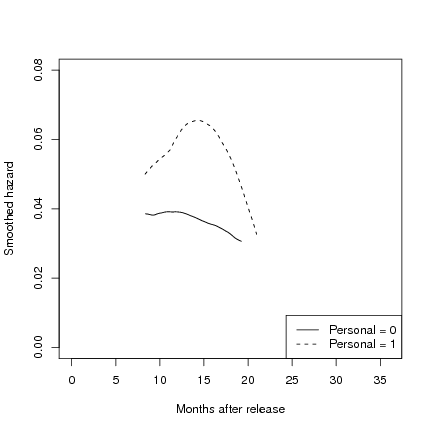Figure 14.2, page 508. To generate the first in this set of graphs, we created a step function from the log of the cumulative hazard calculated in the previous example.

 ```> l.hat.0 <- log(h.hat.0) > l.hat.1 <- log(h.hat.1) > log.h.steps.0<- stepfun(time.0, c(l.hat.0, l.hat.0)) > log.h.steps.1<- stepfun(time.1, c(l.hat.1, l.hat.1)) > par(mfrow=c(1,1)) > plot(log.h.steps.0, do.points = FALSE, xlim = c(0, 36), ylim = c(-6.0,1), ylab = "Log(Cumulative Hazard)", xlab = "Months after release", main = "") > lines(log.h.steps.1, do.points = FALSE, xlim = c(0,36), lty = 2) > points(c(-5, 36), c(0,0), type = "l") > legend("bottomright", c("Personal = 0", "Personal = 1"), lty = c(1, 2)) ```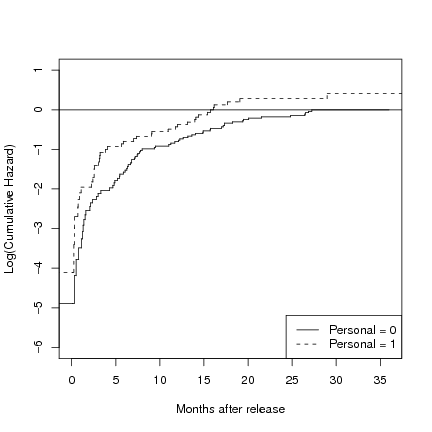To generate the second and third graphs in this set, we first ran a Cox model with personal as a predictor. We then created one data frame where personal is 0 and one data frame where personal is 1 and used the model to obtain estimates of the hazard rate for each value of personal (using the proportional hazards assumption). We then plotted the fitted curves from the model and overlaid the non-parametric estimates for the log cumulative hazard at the times of events on the second graph. The third graph is based on the cumulative hazard rather than the log cumulative hazard.

 ```> attach(rearrest) > f14.2 <- coxph(Surv(months, abs(censor - 1))~personal, method = "efron") > personal.0 <- data.frame(personal=0) > personal.1 <- data.frame(personal=1) > s.base <- survfit(f14.2, newdata = personal.0, type = "kaplan-meier") > s.personal <- survfit(f14.2, newdata = personal.1, type = "kaplan-meier") > h.base <- -log(s.base\$surv) > h.personal <- -log(s.personal\$surv) > l.h.base <- log(h.base) > l.h.personal <- log(h.personal) > plot(s.base\$time, l.h.base, type = "l", lty = 1, ylim = c(-6, 1), xlab = "Months after release", ylab = "Cumulative Hazard") > points(s.personal\$time, l.h.personal, type = "l", lty = 2) > points(time.0, l.hat.0, pch = 3) > points(time.1, l.hat.1, pch = 4) > legend("bottomright", c("Personal = 0", "Personal = 1"), lty = c(1, 2)) ```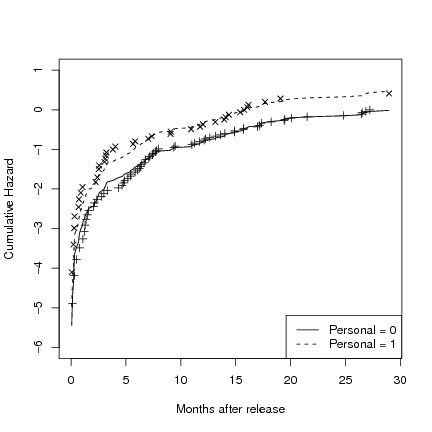```> plot(s.base\$time, h.base, type = "l", lty = 1, ylim = c(0,1.5), xlab = "Months after release", ylab = "Cumulative Hazard") > points(s.personal\$time, h.personal, type = "l", lty = 2) > points(time.0, h.hat.0, pch = 3) > points(time.1, h.hat.1, pch = 4) > legend("bottomright", c("Personal = 0", "Personal = 1"), lty = c(1, 2)) ```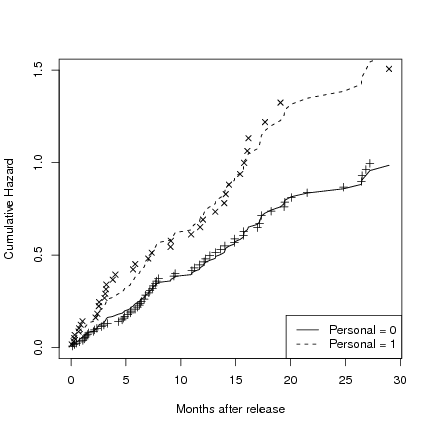Table 14.1, page 525. To generate the parameter estimates and model statistics seen in this table, we ran Cox models with the predictors specified in the table.

 ```> tab14.1A <- coxph(Surv(months, abs(censor - 1))~personal) > summary(tab14.1A) ```

 ```Call: coxph(formula = Surv(months, abs(censor - 1)) ~ personal) n= 194 coef exp(coef) se(coef) z Pr(>|z|) personal 0.4787 1.6140 0.2025 2.364 0.0181 * --- Signif. codes: 0 ‘***’ 0.001 ‘**’ 0.01 ‘*’ 0.05 ‘.’ 0.1 ‘ ’ 1 exp(coef) exp(-coef) lower .95 upper .95 personal 1.614 0.6196 1.085 2.4 Rsquare= 0.027 (max possible= 0.994 ) Likelihood ratio test= 5.32 on 1 df, p=0.02104 Wald test = 5.59 on 1 df, p=0.01807 Score (logrank) test = 5.69 on 1 df, p=0.01702 ```

 ```> tab14.1B <- coxph(Surv(months, abs(censor - 1))~property) > summary(tab14.1B) ```

 ```Call: coxph(formula = Surv(months, abs(censor - 1)) ~ property) n= 194 coef exp(coef) se(coef) z Pr(>|z|) property 1.1946 3.3022 0.3493 3.42 0.000626 *** --- Signif. codes: 0 ‘***’ 0.001 ‘**’ 0.01 ‘*’ 0.05 ‘.’ 0.1 ‘ ’ 1 exp(coef) exp(-coef) lower .95 upper .95 property 3.302 0.3028 1.665 6.548 Rsquare= 0.08 (max possible= 0.994 ) Likelihood ratio test= 16.2 on 1 df, p=0.00005684 Wald test = 11.7 on 1 df, p=0.000626 Score (logrank) test = 13.14 on 1 df, p=0.0002896 ```

 ```> tab14.1C <- coxph(Surv(months, abs(censor - 1))~cage) > summary(tab14.1C) ```

 ```Call: coxph(formula = Surv(months, abs(censor - 1)) ~ cage) n= 194 coef exp(coef) se(coef) z Pr(>|z|) cage -0.06813 0.93414 0.01563 -4.359 0.0000131 *** --- Signif. codes: 0 ‘***’ 0.001 ‘**’ 0.01 ‘*’ 0.05 ‘.’ 0.1 ‘ ’ 1 exp(coef) exp(-coef) lower .95 upper .95 cage 0.9341 1.071 0.906 0.9632 Rsquare= 0.112 (max possible= 0.994 ) Likelihood ratio test= 22.97 on 1 df, p=0.000001646 Wald test = 19 on 1 df, p=0.00001306 Score (logrank) test = 19.18 on 1 df, p=0.00001191 ```

 ```> tab14.1D <- coxph(Surv(months, abs(censor - 1))~personal + property + cage) > summary(tab14.1D) ```

 ```Call: coxph(formula = Surv(months, abs(censor - 1)) ~ personal + property + cage) n= 194 coef exp(coef) se(coef) z Pr(>|z|) personal 0.56914 1.76674 0.20521 2.773 0.00555 ** property 0.93579 2.54922 0.35088 2.667 0.00765 ** cage -0.06671 0.93546 0.01678 -3.976 0.00007 *** --- Signif. codes: 0 ‘***’ 0.001 ‘**’ 0.01 ‘*’ 0.05 ‘.’ 0.1 ‘ ’ 1 exp(coef) exp(-coef) lower .95 upper .95 personal 1.7667 0.5660 1.1817 2.6415 property 2.5492 0.3923 1.2816 5.0708 cage 0.9355 1.0690 0.9052 0.9667 Rsquare= 0.182 (max possible= 0.994 ) Likelihood ratio test= 38.96 on 3 df, p=0.00000001770 Wald test = 29.02 on 3 df, p=0.000002214 Score (logrank) test = 30.3 on 3 df, p=0.000001195 ```

Table 14.2, page 533. To generate this table, we wrote a function risk.score that takes an ID as an argument and returns a vector containing the information for the given ID (including the risk score). We then called this function for the set of IDs that appear in the table and combined the vectors of information from each function call into a table.

 ```> risk.score <- function(ID.num){ temp <- subset(rearrest, id==ID.num) log.score <- tab14.1D\$coef*temp\$personal + tab14.1D\$coef*temp\$property + tab14.1D\$coef*temp\$cage score <- exp(log.score) day <- temp\$months * (365/12) table.row <- cbind(temp\$id, temp\$personal, temp\$property, temp\$cage, score, day, temp\$months, temp\$censor) colnames(table.row) <- c("ID", "personal", "property", "centered.age", "risk.score", "day", "months", "censor") rownames(table.row) <- c() return(table.row)} > tab.14.2 <- rbind( risk.score(22), risk.score(8), risk.score(187), risk.score(26), risk.score(5), risk.score(130), risk.score(106), risk.score(33)) > tab.14.2 ```

 ``` ID personal property centered.age risk.score [1,] 22 0 0 0.2577249 0.9829532 [2,] 8 1 1 22.4507434 1.0071815 [3,] 187 1 0 -7.2001806 2.8561843 [4,] 26 0 1 -7.3014811 4.1491205 [5,] 5 1 1 -7.1645886 7.2637815 [6,] 130 0 1 22.3905107 0.5723740 [7,] 106 0 0 16.2029679 0.3392704 [8,] 33 1 0 27.0612841 0.2904806 day months censor [1,] 51.96441 1.7084189 1 [2,] 18.98700 0.6242300 1 [3,] 1095.00000 36.0000000 1 [4,] 71.95072 2.3655031 0 [5,] 8.99384 0.2956879 0 [6,] 485.66735 15.9671458 1 [7,] 355.75633 11.6960986 0 [8,] 84.94182 2.7926078 1 ```

Figure 14.4, page 538. To generate these plots, we first reran the fourth model from table 14.1 and created two data frames: one representing the baseline (all predictors equal to zero) and one representing the average (all predictors equal to their sample means). We then used the model to predict survival and cumulative hazard rates for both the baseline and average case and plotted the results in the first four graphs. For the fifth and sixth graphs, we again used the smooth function described earlier in this page to generate kernel smoothed values of the hazard function.

 ```> tab14.1D <- coxph(Surv(months, abs(censor - 1))~personal + property + cage) > base <- data.frame(personal=0, property=0, cage=0) > ss <- survfit(tab14.1D) > ss.base <- survfit(tab14.1D, newdata = base) > avg <- data.frame(personal=mean(personal), property=mean(property), cage=0) > ss.avg <- survfit(tab14.1D, newdata = avg) > par(mfrow=c(1,2)) > plot(ss.base\$time, ss.base\$surv, type = "l", ylim = c(0,1), xlab = "Months after release", ylab = "Estimated Survival") > points(ss.base\$time, ss.base\$surv, pch = 20) > plot(ss.avg\$time, ss.avg\$surv, type = "l", ylim = c(0,1), xlab = "Months after release", ylab = "Estimated Survival") > points(ss.avg\$time, ss.avg\$surv, pch = 20) ```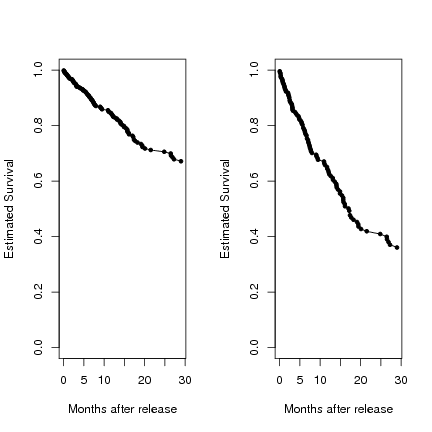```> plot(ss.base\$time, -log(ss.base\$surv), type = "l", ylim = c(0,1.5), xlab = "Months after release", ylab = "Estimated Cumulative Hazard") > points(ss.base\$time, -log(ss.base\$surv), pch = 20) > plot(ss.avg\$time, -log(ss.avg\$surv), type = "l", ylim = c(0,1.5), xlab = "Months after release", ylab = "Estimated Cumulative Hazard") > points(ss.avg\$time, -log(ss.avg\$surv), pch = 20) ```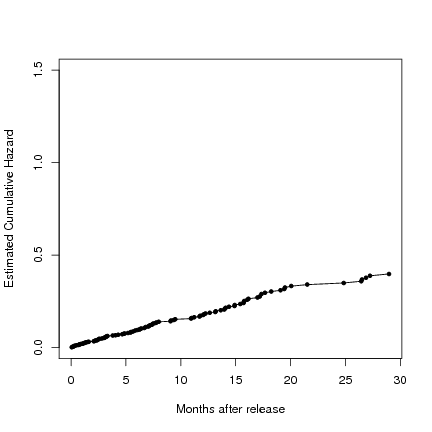```> smooth.base <- smooth(8, ss.base\$time, ss.base\$surv) > smooth.avg<- smooth(8, ss.avg\$time, ss.avg\$surv) > plot(smooth.base\$x, smooth.base\$y, type = "l", xlim = c(0, 36), ylim = c(0, .08), xlab = "Months after release", ylab = "Smoothed hazard") > plot(smooth.avg\$x, smooth.avg\$y, type = "l", xlim = c(0, 36), ylim = c(0, .08), xlab = "Months after release", ylab = "Smoothed hazard") ```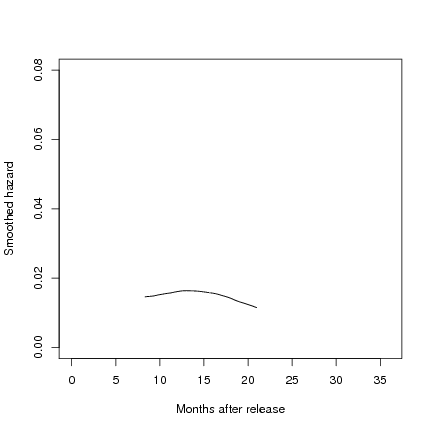Figure 14.5, page 541.To generate these plots, we first reran the fourth model from table 14.1 and created four data frames, all with age variable set to its mean (zero, since age is centered). One data frame had personal and property values both set to 0, one data frame had personal set to 1 and property to 0, one data frame had property set to 1 and personal set to 0, and the fourth data frame had both personal and property set to zero. We then used the model to predict survival (first graph), cumulative hazard (second graph), and log cumulative hazard (third graph) for each of the four scenarios represented in a data frame and plotted each set of estimates.

 ```> tab14.1D <- coxph(Surv(months, abs(censor - 1))~personal + property + cage) > base <- data.frame(personal=0, property=0, cage=0) > personal.only <- data.frame(personal=1, property=0, cage=0) > property.only <- data.frame(personal=0, property=1, cage=0) > both <- data.frame(personal=1, property=1, cage=0) > s.base <- survfit(tab14.1D, newdata = base) > s.personal <- survfit(tab14.1D, newdata = personal.only) > s.property <- survfit(tab14.1D, newdata = property.only) > s.both <- survfit(tab14.1D, newdata = both) > par(mfrow=c(1,1)) > plot(s.base\$time, s.base\$surv, type = "l", lty = 1, ylim = c(0,1), xlab = "Months after release", ylab = "Estimated Survival") > points(s.personal\$time, s.personal\$surv, type = "l", lty = 2) > points(s.property\$time, s.property\$surv, type = "l", lty = 3) > points(s.both\$time, s.both\$surv, type = "l", lty = 4) > legend("bottomleft", c("Personal = 0, Property = 0", "Personal = 1, Property = 0", "Personal = 0, Property = 1", "Personal = 1, Property = 1"), lty = c(1, 2, 3, 4)) ```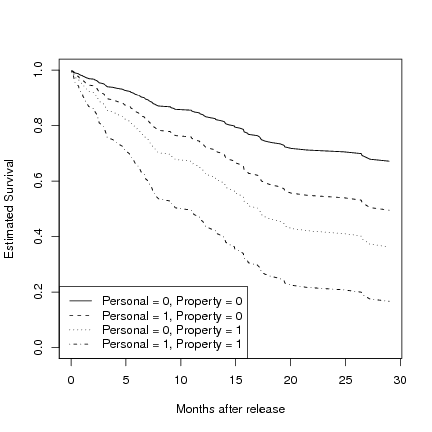```> h.base <- -log(s.base\$surv) > h.personal <- -log(s.personal\$surv) > h.property <- -log(s.property\$surv) > h.both <- -log(s.both\$surv) > plot(s.base\$time, h.base, type = "l", lty = 1, ylim = c(0,2), xlab = "Months after release", ylab = "Estimated Cumulative Hazard") > points(s.personal\$time, h.personal, type = "l", lty = 2) > points(s.property\$time, h.property, type = "l", lty = 3) > points(s.both\$time, h.both, type = "l", lty = 4) > legend("topleft", c("Personal = 0, Property = 0", "Personal = 1, Property = 0", "Personal = 0, Property = 1", "Personal = 1, Property = 1"), lty = c(1, 2, 3, 4)) ```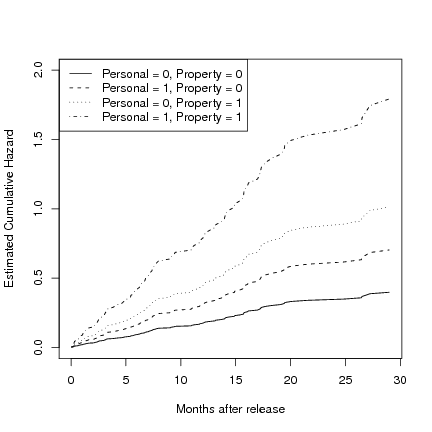```> l.h.base <- log(h.base) > l.h.personal <- log(h.personal) > l.h.property <- log(h.property) > l.h.both <- log(h.both) > plot(s.base\$time, l.h.base, type = "l", lty = 1, ylim = c(-7,1), xlab = "Months after release", ylab = "Log(Cumulative Hazard)") > points(s.personal\$time, l.h.personal, type = "l", lty = 2) > points(s.property\$time, l.h.property, type = "l", lty = 3) > points(s.both\$time, l.h.both, type = "l", lty = 4) > legend("bottomright", c("Personal = 0, Property = 0", "Personal = 1, Property = 0", "Personal = 0, Property = 1", "Personal = 1, Property = 1"), lty = c(1, 2, 3, 4)) ```